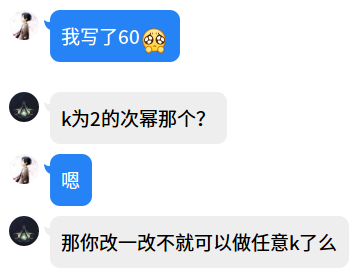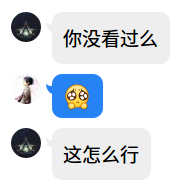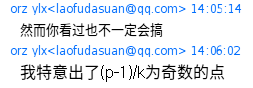# HNOI 2019 简要题解

code

code

code

## Day2T1 校园旅行

（不知道怎么想的）考虑减少边数。对于所有连接同色点的边，每一个连通块内只需要保留一棵生成树即可得到与原图相同的结果。特别的，若该连通块不是二分图，则需要添加一条自环。对于所有连接异色点的边做同样的事情，这样总边数就降到了$O(n)$级别，再套用上面的做法即可。

code

## Day2T2 白兔之舞

$b_t=\sum_{i=0}^L[i\%k=t]\binom Li(A^i)_{x,y}$

$b_t=\frac{1}{k}\sum_{i=0}^L\sum_{j=0}^{k-1}\omega_k^{j(i-t)}\binom Li(A^i)_{x,y}\\=\frac{1}{k}\sum_{j=0}^{k-1}\omega_k^{-jt}\sum_{i=0}^L\binom Li\omega_k^{ij}(A^i)_{x,y}\\=\frac{1}{k}\sum_{j=0}^{k-1}\omega_k^{-jt}((\omega_k^jA+I)^L)_{x,y}$

$a_j=((\omega_k^jA+I)^L)_{x,y}$，然后原式（差不多）就变成了

$b_t=\sum_{j=0}^{k-1}\omega_k^{jt}a_j$$b_t=\sum_{j=0}^{k-1}\frac{\omega_k^{\frac{(j+t)^2}{2}}}{\omega_k^{\frac{j^2}{2}}\omega_k^{\frac{t^2}{2}}}a_j$$jt=\binom{j+t}{2}-\binom j2-\binom t2$

$b_t=\sum_{j=0}^{k-1}\frac{\omega_k^{\binom{j+t}{2}}}{\omega_k^{\binom{j}{2}}\omega_k^{\binom{t}{2}}}a_j$

$MTT$实现卷积即可，复杂度依旧为$O(3^3k\log L+k\log k)$

code

## Day2T3 序列

code

posted @ 2019-04-09 21:55  租酥雨  阅读(2145)  评论(13编辑  收藏